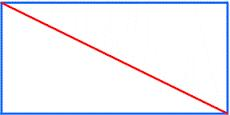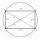# Rectangle 3-4-5

The sides of the rectangle are in a ratio of 3:4. The length of the rectangle diagonal is 20 cm. Calculate the content of the rectangle.

S =  192 cm2

### Step-by-step explanation:Did you find an error or inaccuracy? Feel free to write us. Thank you!Tips to related online calculators
Check out our ratio calculator.
Pythagorean theorem is the base for the right triangle calculator.
Do you want to convert length units?

#### You need to know the following knowledge to solve this word math problem:

We encourage you to watch this tutorial video on this math problem:

## Related math problems and questions:

• The sidesThe sides of the rectangle are in a ratio of 3: 5 and its circumference measures 72 cm. Calculate: a) the size of both sides of the rectangle b) the area of the rectangle c) the length of the diagonals
• Isosceles trapezoidCalculate the content of an isosceles trapezoid whose bases are at ratio 5:3, the arm is 6cm long and it is 4cm high.
• Rhombus and diagonalsThe rhombus area is 150 cm2, and the ratio of the diagonals is 3:4. Calculate the length of its height.
• RectangleThe length of the rectangle are in the ratio 5:12 and the circumference is 238 cm. Calculate the length of the diagonal and area of rectangle.
• ISO triangleCalculate the area of an isosceles triangle KLM if its sides' length is in the ratio k:l:m = 4:4:3 and has perimeter 377 mm.
• Rectangle diagonalsIt is given a rectangle with an area of 24 cm2 a circumference of 20 cm. The length of one side is 2 cm larger than the length of the second side. Calculate the length of the diagonal. Length and width are yet expressed in natural numbers.
• RhombusThe rhombus has diagonal lengths of 4.2cm and 3.4cm. Calculate the length of the sides of the rhombus and its height
• Diagonal to areaCalculate the area of a rectangle in which the length of the diagonal is 10 cm.
• Rectangle 35Find the area of a rectangle when the diagonal is equal to 30 cms and the width is double the length.
• Rectangle diagonalThe rectangle, one side of which is 5 cm long, is divided by a 13 cm diagonal into two triangles. Calculate the area of one of these triangles in cm2.
• Ratio of sidesCalculate the area of a circle with the same circumference as the circumference of the rectangle inscribed with a circle with a radius of r 9 cm so that its sides are in ratio 2 to 7.
• Equilateral triangleThe equilateral triangle has a 23 cm long side. Calculate its content area.
• Ratio of edgesThe dimensions of the cuboid are in a ratio 3: 1: 2. The body diagonal has a length of 28 cm. Find the volume of a cuboid.
• A rectangle 2A rectangle has a diagonal length of 74cm. Its side lengths are in ratio 5:3. Find its side lengths.
• TrapezoidArea of trapezoid is 135 cm2. Sides a, c and height h are in a ratio 6:4:3. How long are a,c and h? Make calculation...
• Rectangle - desc circleLength of the sides of the rectangle are at a ratio 1: 3 . Radius of the circle circumscribed to rectangle is 10 cm. Calculate the rectangle's perimeter.
• Rectangular gardenThe sides of the rectangular garden are in ratio 1: 2. The diagonal has a length of 20 meters. Calculate the area and perimeter of the garden.